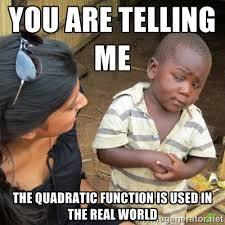4 years ago
athomson
Save
Edit
Host a game
Live GameLive
Homework
Solo Practice
Practice10 QuestionsShow answers
• Question 1
120 seconds
Q. Identify the 'a' value: y = 16x2 -8x -24
16
8
-8
-24
• Question 2
120 seconds
Q. Identify the 'b' value: y = 16x2 -8x -24
16
-8
8
-24
• Question 3
120 seconds
Q. Identify the 'c' value: y = 16x2 -8x -24
16
-8
24
-24
• Question 4
300 seconds
Q. b2  would equal: y = 2x2 -x -3
2
-1
1
-3
• Question 5
300 seconds
Q. 4ac would equal:  y = 2x2 -x -3
-12
8
-24
-1
• Question 6
300 seconds
Q. b2 - 4ac would equal: y = 2x2 -x -3
25
-23
-25
0
• Question 7
300 secondsQ.
A
B
C
D
• Question 8
300 secondsQ.
A
B
C
D
• Question 9
300 secondsQ.
A
B
C
D
• Question 10
300 secondsQ.